0
Thanks

A few words of thanks would be greatly appreciated.

# Excel - How to translate formulas (from French to English)

## Excel - How to translate formulas (from French to English)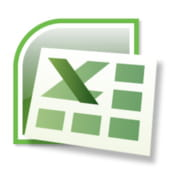## Introduction

It is not always easy to translate complex formulas, especially if they are written in French. In this trick we will make use of the VBA/Excel interface ( but without adding code any to your workbook) to translate formulas.

## Procedure:

• Enter your formula in the Excel sheet,
• Validate this formula,
• Select the cell that contains your formula
• Press ALT + F11,
• Press Ctrl + G,
• In the Run window, type:? ActiveCell.Formula
• Press Enter

The formula has been translated to English ...

## Example:

1-Enter your formula in the Excel sheet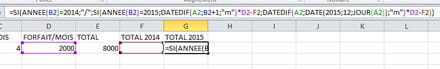2- Confirm this formula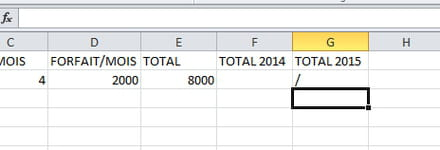3. Select the cell that contains your formula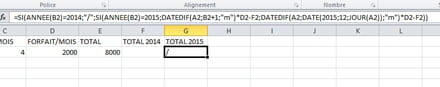4- Press ALT + F11, followed by CTRL + G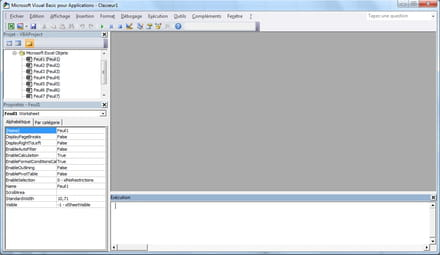6- In the Run window, type: ? ActiveCell.Formula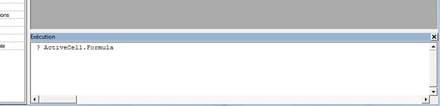7- Press Enter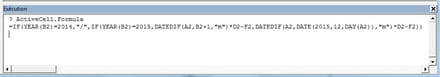0
Thanks

A few words of thanks would be greatly appreciated.